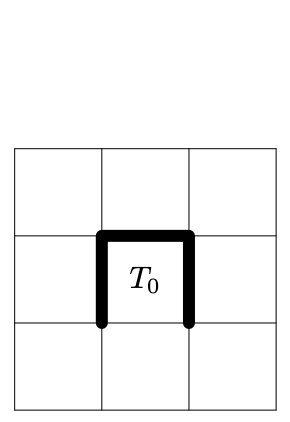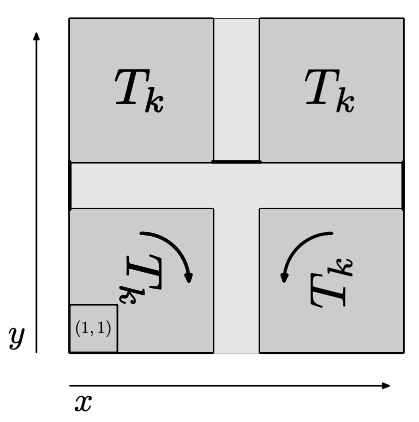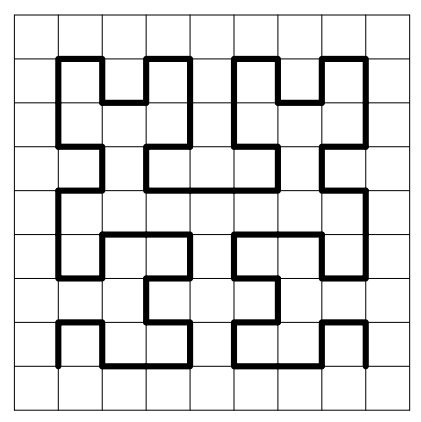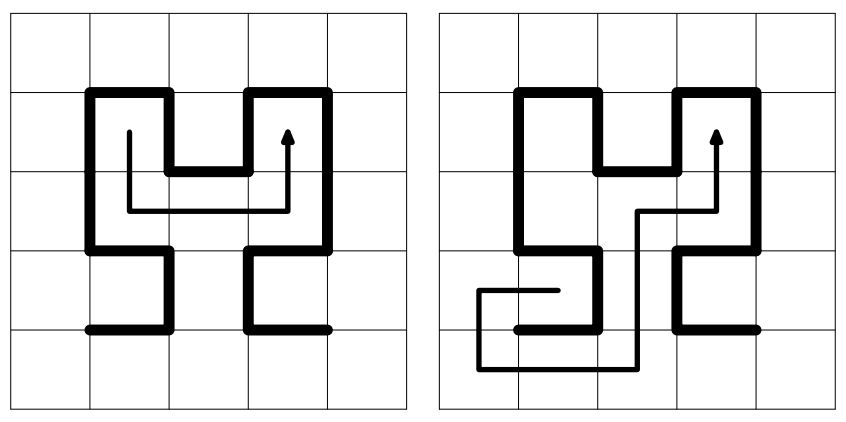시간 제한 메모리 제한 제출 정답 맞은 사람 정답 비율
5 초 256 MB 1 1 1 100.000%

## 문제

You are playing a computer game "Hilbert's Maze". The point of the game is to find a way out of various mazes. The last level of the game features the most tricky maze, which you find very difficult to escape from. Frustrated by the maze's complexity, you decided to put your programming skills to use.

The maze can be described as a rectangular field divided into identical square cells, with walls along some borders of the cells. Standing in a cell, you can move to any of the adjacent cells (two cells are adjacent if they share a border segment) if there is no wall in your way.

The particular maze you're going to escape from can be constructed using the following recursive pattern: starting from the basic maze $T_0$ (figure 1), you apply the following transformation (figure 2) $k$ times to obtain the resulting maze $T_k$. You've introduced the coordinate system as shown on figure 2, with the cell in the lower left corner having coordinates $(1, 1)$. For example, the maze $T_2$ is shown on figure 3.Figure 1 Figure 2 Figure 3

It is possible to escape the boundaries of the maze (that is, the square with corner cells $(1, 1)$ and $(2^{k+1} - 1, 2^{k+1} - 1)$); there are no walls outside the boundaries, and you are free to move along the border or as far as you like from the maze. You have to find the shortest way from some starting cell to some target cell. The starting and target cells are generated randomly each time you try, so you would like to write a program that finds the shortest path for many instances of the problem.

## 입력

The first line contains an integer $T$ ($1 \leq T \leq 3 \cdot 10^5$) --- the number of test cases.

Next $T$ lines contain descriptions of the test cases. The $i$-th of these lines contains five space-separated integers $k$, $x_s$, $y_s$, $x_t$, $y_t$ ($0 \leq k \leq 30$, $1 \leq x_s, y_s, x_t, y_t \leq 2^{k+1} - 1$) --- the number of transformations used to construct the maze and the coordinates of the starting and the target cells. The cells may coincide.

## 출력

Output $T$ lines; on the $i$-th of these lines print a single integer --- the smallest number of moves needed to get from the starting cell to the target cell. It is guaranteed that a way between the cells exists.

## 예제 입력 1

3
0 1 1 1 1
1 1 3 3 3
1 1 1 3 3


## 예제 출력 1

0
4
8


## 힌트

The second and third samples are illustrated below.